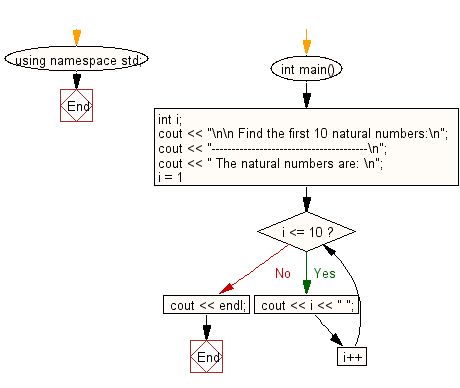﻿ C++ Exercises: Find the first 10 natural numbers - w3resource

# C++ Exercises: Find the first 10 natural numbers

## C++ For Loop: Exercise-1 with Solution

Write a program in C++ to find the first 10 natural numbers.

Pictorial Presentation:Sample Solution :-

C++ Code :

``````#include <iostream>
using namespace std;
int main()
{
int i;
cout << "\n\n Find the first 10 natural numbers:\n";
cout << "---------------------------------------\n";
cout << " The natural numbers are: \n";
for (i = 1; i <= 10; i++)
{
cout << i << " ";
}
cout << endl;
}
``````

Sample Output:

``` Find the first 10 natural numbers:
---------------------------------------
The natural numbers are:
1 2 3 4 5 6 7 8 9 10
```

Flowchart:C++ Code Editor: